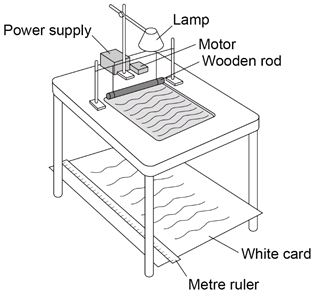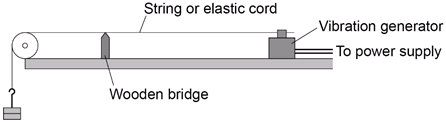# Investigate Properties of a Wave Required Practical 0

## Experimental Introduction

There are two practicals that you need to know. The first is observing water waves in a ripple tank with the second observing on a stretched string or chord.

## Method 1

You are provided with the following:

1. Ripple tank plus accessories
2. Suitable low voltage power supply
3. Metre ruler

Read these instructions carefully before you start work.

1. Set up the ripple tank. A large sheet of white card or paper needs to be on the floor under the tank.1. Pour water to a depth of about 5 mm into the tank.

2. Adjust the height of the wooden rod so that it just touches the surface of the water.

3. Switch on both the overhead lamp and the electric motor.

4. Adjust the speed of the motor. Low frequency water waves need to be produced.

5. Adjust the height of the lamp. The pattern needs to be clearly seen on the card on the floor.

6. Place a metre ruler at right angles to the waves shown in the pattern on the card.

Measure across as many waves as possible. Then divide that length by the number of waves. This gives the wavelength of the waves.

1. Count the number of waves passing a point in the pattern over a given time (say 10 seconds).

Then divide the number of waves counted by 10. This gives the frequency of the waves.

1. Calculate the speed of the waves using the equation:

wave speed = frequency wavelength

## Method 2

You are provided with the following:

1. Vibration generator
2. Suitable power supply (variable frequency)
3. Suitable string or elasticated cord
4. Set of 100 g masses and hanger
5. Set of 10 g masses and hanger
6. Wooden bridge
7. Pulley on a clamp

Read these instructions carefully before you start work

1. Set up the apparatus as shown.1. Switch on the vibration generator. The string (or elasticated cord) should start to vibrate.

2. A clear wave pattern needs to be seen. To do this, adjust the tension in the string or move the wooden bridge to adjust the length of the string.

The waves should look like they are stationary.

1. Use a metre ruler to measure across as many half wavelengths as possible (a half wavelength is one loop).

Then divide the total length by the number of half waves. Multiplying this number by two will give the wavelength.

1. The frequency is the frequency of the power supply.

2. Calculate the speed of the wave using the equation:

wave speed = frequency wavelength